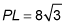##### Geometry: 1,001 Practice Problems For Dummies (+ Free Online Practice)In geometry, special right triangles are great to work with because the ratio of their sides will always be the same, making calculations easier. The two special triangles you need to know are the isosceles (or 45-45-90) and 30-60-90 right triangles.

You can use your knowledge of special right triangles to answer the following questions.

## Practice questions

1. The length of the altitude of an equilateral triangle is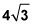Find the length of a side of the triangle.

2. In isosceles right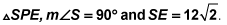Rectangle PLCE,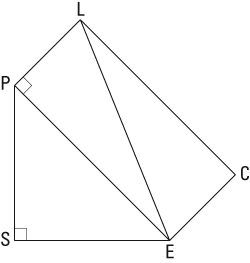with diagonal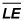drawn forms a 30-degree angle at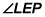Find the length of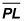1. 8

An equilateral triangle is a triangle with three congruent sides and three congruent angles. Each angle therefore measures 60 degrees. When you draw the altitude, it creates two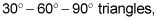as in the following figure.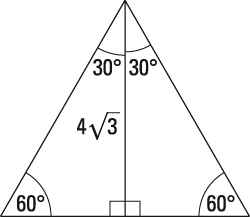The pattern of the sides of a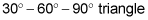is as follows: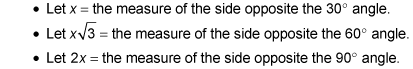The altitude is the side opposite the 60-degree angle; therefore,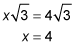A side of the equilateral triangle is opposite the 90-degree angle, so it's equal to 2x; therefore, a side of the triangle equals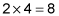2.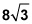The pattern of the sides of an isosceles right triangle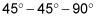is as follows: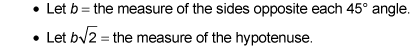In this problem, the side opposite the 45-degree angle is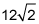therefore,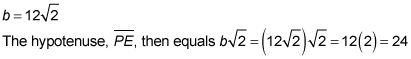As shown in the figure,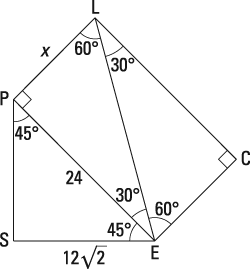diagonal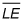divides this rectangle into two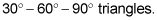The pattern of the sides of ais as follows: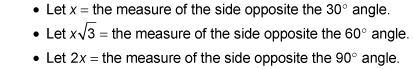Line segment PE, measuring 24, is the side opposite the 60-degree angle, so it's equal to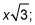therefore,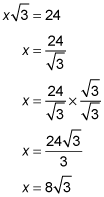Line segment PL is the side opposite the 60-degree angle, so it equals x. Therefore,